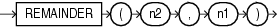## REMAINDER

SyntaxDescription of the illustration remainder.eps

Purpose

`REMAINDER` returns the remainder of `n2` divided by `n1`.

This function takes as arguments any numeric data type or any nonnumeric data type that can be implicitly converted to a numeric data type. Oracle determines the argument with the highest numeric precedence, implicitly converts the remaining arguments to that data type, and returns that data type.

The `MOD` function is similar to `REMAINDER` except that it uses `FLOOR` in its formula, whereas `REMAINDER` uses `ROUND`. Refer to MOD.

Table 2-8 for more information on implicit conversion and for information on numeric precedence

• If `n1` = 0 or `n2` = infinity, then Oracle returns

• An error if the arguments are of type `NUMBER`

• `NaN` if the arguments are `BINARY_FLOAT` or `BINARY_DOUBLE`.

• If `n1` != 0, then the remainder is `n2` - (`n1`*`N`) where `N` is the integer nearest `n2`/`n1`. If `n2`/`n1` equals `x.5`, then `N` is the nearest even integer.

• If `n2` is a floating-point number, and if the remainder is 0, then the sign of the remainder is the sign of `n2`. Remainders of 0 are unsigned for `NUMBER` values.

Examples

Using table `float_point_demo`, created for the `TO_BINARY_DOUBLE` "Examples", the following example divides two floating-point numbers and returns the remainder of that operation:

```SELECT bin_float, bin_double, REMAINDER(bin_float, bin_double)
FROM float_point_demo;

BIN_FLOAT BIN_DOUBLE REMAINDER(BIN_FLOAT,BIN_DOUBLE)
---------- ---------- -------------------------------
1.235E+003 1.235E+003                      5.859E-005```